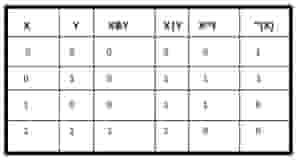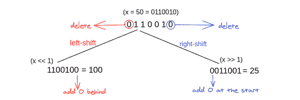# Algorithms 101: the basics of Bit Manipulation explained

Jan 21, 2021 - 6 min readJerry EjonaviComputers do not understand words and numbers the way we do. All the data it receives are encoded at the lowest level to a series of zeros and ones. (0 and 1), and this is the only way it makes sense of any command it’s given. This series of 0 and 1 are known as bits.

This tutorial is intended to be an introduction to Bit algorithms for programmers. We’ll start with learning about Bits. Then, we’ll move on to explore a few algorithms for manipulating Bits. Finally, we’ll wrap things up with some common interview questions you can practice with in Python or Java.

Today, we will go over:

## Introduction to Bits

Bit stands for Binary Digit, a basic and smallest unit of data in a computer. Binary representation is based on 1s and 0s. A Bit represents a logical state with only 2 boolean values, either 0 or 1, as they are a 2 base number. They are the lowest level of language used in machines.

Note: We read binary number from the right to left.

A binary digit

Working with bytes is normal for any programmer. This includes manipulating any data type that is made up of bytes, such as ints, floats, doubles and data structures. In order to encode, decode, or compress files, we extract the data at bit level.

Bit manipulation is the act of algorithmically manipulating bits using bit-level (bitwise) operations. These bitwise operations are the heart of bit manipulation. They are primitive, fast actions that are used in improving the efficiency of a program.

## Basics of Bit manipulation (Operators)A table denoting bitwise operators

Terminology: A bitmask is the data used for bitwise operations, particularly in a bit field. Using a mask, bits can be set either on/off or vice versa in a single bitwise operation.

The bitwise operations you can perform on individual Bits require any of the following operators:

### & (And operator)

The & operator takes two equal-length bit patterns as parameters. The two bit integers are compared. If the bits in the compared positions of the bit patterns are 1, then the resulting bit is 1. If not, it is 0.

For example; take two bit values $X$ and $Y$, where $X= 10 = (1010)_2$ , and $Y = 9 = (1001)_2$, let’s attempt to find $X$ & $Y$.

To solve this, you take the first pair of values from the right and compare, since $0 != 1$ the result is $0$. The second and third pairs do not match so we get 0 from them. The final bit pair has matching sets of 1, so the result is 1. Our final value is 1000, or 8.

X & Y = (1010)_2 & $(1001)_2 = (1000)_2 = 8$

### | (Or operator)

The | operator takes two equal-length bit patterns as parameters, if both bits in the compared position are 0, the resulting bit is zero. If not, it is 1.

For example: take two bits $X$ and $Y$, where $X = 10 = (1010)_2$ , and $Y = 9 = (1001)_2$, let’s attempt to find $X | Y$.

$X | Y = (1010)_2$ | $(1001)_2 = (1011)_2 = 11$

### Keep the learning going.

Learn Bit manipulation without scrubbing through videos or documentation. Educative’s text-based courses are easy to skim and feature live coding environments, making learning quick and efficient. In this course, you will learn

Master Solving Problems using Bit Manipulation

### ^ Exclusive-or, Xor Operator

The ^ operator (also known as the XOR operator) stands for Exclusive Or. Here, if bits in the compared position do not match its resulting bit is 1. This is efficient in checking for duplicates.

$X= 10=(1010)_2$ , $Y = 9=(1001)_2$

$X ^ Y = (1010)_2$ ^ $(1001)_2 = (0011)2 = 3$

### ~ (Not Operator)

The ~ operator flips the bits of a number. This means that if the ith bit is 0, it changes to 1, while 1 would flip to zero. If ~ is passed a positive value $X$, it returns $-x -1$, i.e $|x| - 1$. Let’s take an example:

$100 = 01100100;$ $-101 = 10011011$

### Shift OperatorsBitwise shift operators

Left-Shift (<<)

The left shift operator is denoted by the double left arrow key (<<). It is used to shift bits to the left: bits towards the left are removed and a zero is added to the right for every bit removed.

The number of times a bit is shifted left is denoted by the variable on the right in the below diagram:

$1001 << n$

Every bit shift left effectively doubles the value of the original bits. Here are a few examples to help you see how it works:

$1 << 1 = 2 = 21$

$1 << 2 = 4 = 22$

$1 << 3 = 8 = 23$

$1 << 4 = 16 = 24$

$1 << n = 2n$

Right-Shift (>>)

The right shift operator is denoted by the double right arrow key (<<). It works by adding copies of the bit at the leftmost end in from the left, while removing the bits at the right. The resulting number is usually half of the initial number.

$1011 >> 1 = 1101$

$0011 >> 2 = 0000$

## Application of BIT Operators

• Bit operations are used for optimization of embedded systems.
• The Exclusive-or operator can be used to confirm the integrity of a file, making sure it has not been corrupted, especially after it has been in transit.
• Bitwise operations are used in Data encryption and compression.
• Bits are used in the area of networking, framing the packets of numerous bits which are sent to another system generally through any type of serial interface.
• Digital Image Processors use bitwise operations to enhance image pixels and to extract different sections of a microscopic image.

## What to learn next

That’s it for Bit manipulation. Nice going! Do you know that bit manipulation can be used to solve common programming challenges that can come up during interviews?

Some of these problems include:

• Checking for odd and even numbers in a sequence
• Counting set bits in an integer
• Multiplying two numbers using bitwise operators (Russian Peasant)
• Generating n-bit Gray Codes
• Detecting if two integers have opposite signs
• Adding 1 to a given number

If you want to start practicing these concepts, check out Educative’s course Master Solving Problems using Bit Manipulation. In this course, you will learn how to solve problems using bit manipulation, a powerful technique that can be used to optimize your algorithmic and problem-solving skills.